### Home > PC3 > Chapter 2 > Lesson 2.2.3 > Problem2-84

2-84.

Calculate the exact length of each side of the triangles. Write your answers in simplified radical form.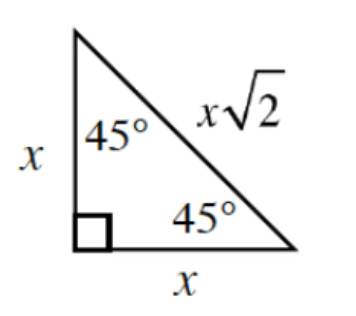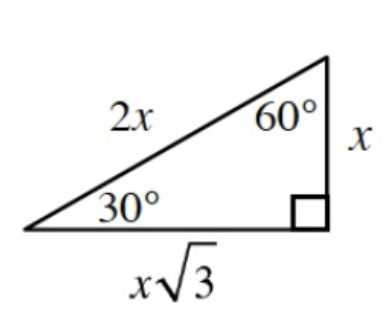1.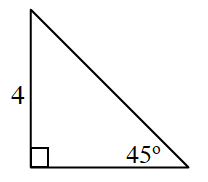$x=4$

1.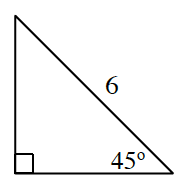$x\sqrt{2}=6$

1.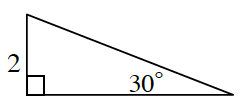$2x=2$